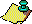Contents Next: The Home Stretch Up: The Beginning of Previous: The Beginning of

The first description of a generalized backtrack search algorithm was in . Let us first define a restricted version of the search problem.
Search problem:
Given a collection of sets of candidates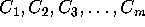and a boolean compatibility function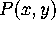defined for all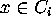and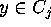, find an m-tuple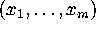with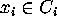such that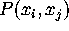is true for all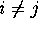.
The m-tuple satisfying the above condition is called a solution. The term compatibility was first introduced by Carter in 1974 . This version is restricted because the compatibility function is only defined on ordered pairs rather than on all k-tuples,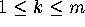.

For example, if we take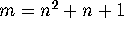and let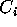be the set of all candidates for column i of the incidence matrix of a projective plane, thencan be defined as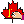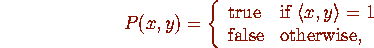where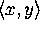denotes the inner product of columns x and y. A solution is then a complete incidence matrix. In a backtrack approach, we generate k-tuples with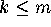. A k-tuples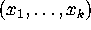is a partial solution at level k ifis true for all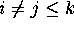. The basic idea of the backtrack approach is to extend a partial solution at level k to one at level k+1, and if this extension is impossible, then to go back to the partial solution at level k-1 and attempt to generate a different partial solution at level k.

A nice way to organize the information inherent in the partial solutions is the backtrack search tree. Here, the empty partial solution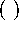is taken as the root, and the partial solution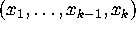is represented as the child of the partial solution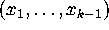. Following the computer science terminology, we often called a partial solution a node. It is often true that the computing cost of processing a node is independent of its level k. Under this assumption, the total computing cost of a search is equal to the number of nodes in the search tree times the cost of processing a node.

The number of nodes in the search tree can often be reduced by using the symmetry or property-preserving operations. If A is the incidence matrix of a projective plane, then the operations of permuting the rows and permuting the columns of A correspond only to reordering the lines and points of the plane. These operations preserve the property of being a projective plane. To see how they reduce the size of a search tree, consider the plane of order 10. There are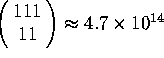choices for the first column, corresponding to the number of ways of placing 11 ones in the 111 rows. By using the row permutations, we can assume that all these ones are placed on the first 11 rows, reducing the number of partial solutions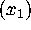from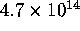to 1. Mathematicians love to use the phrase ``without loss of generality'' to indicate a simplification by symmetry operations. So without loss of generality, the second column has only one choice --- with a one in the first row and the remaining 10 ones in rows 12 to 21. Now, row 1 has nine remaining ones. By permuting columns, we can assume that these remaining ones of row 1 are in columns 3 to 11. Next, by row permutation, the remaining 10 ones of column 3 can be placed in rows 22 to 31. Continuing in this manner, it is not difficult to show that there is only one choice up to column 21. Beyond this point, symmetry operations are difficult to visualize, because they often involve combinations of row and column permutations.

This process of reducing the search tree by using symmetry operations is called isomorph rejection. Two partial solutions are said to be isomorphic if there is a symmetry operation mapping one to the other. To determine whether a solution exists, it is only necessary to extend the non-isomorphic partial solutions, because if a partial solution can be extended to a complete solution, then every other isomorphic partial solution can also be so extended. The testing of whether two partial solutions are isomorphic is called isomorphism testing. The set of symmetry operations mapping a partial solution to itself forms an automorphism group. In the context of projective planes, an automorphism of a complete solution is also called a collineation.

The efficient use of the symmetry operations is one of the most difficult problem in a backtrack search. It is both a blessing and a curse --- a blessing because there is always hope of further optimization and a curse because it is the source of many programming errors. However, following the method of MacWilliams et al., it is clear that the existence question of the plane of order 10 is headed towards a computer-based solution and that symmetry consideration is a necessary tool for efficiency reasons.

In his 1974 Ph. D. thesis, Carter picked up where MacWilliams et al. had left off. He showed that there are six possible starting configurations associated with a codeword of weight 16. By using the computer, he managed to eliminate four of the cases, as well as one subcase of the fifth. He used a total of about 100 hours of computer time, mostly on a CDC 6600 at the Institute for Defense Analyses (IDA) in Princeton, New Jersey, and also on a CDC 7600 at the Lawrence Radiation Laboratories in Berkeley, California. His search investigated about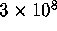nodes, or about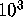nodes per second. This was the situation when we entered the picture. While we knew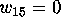, the search for weight 16 codewords was about three-quarter done and the search for weight 12 codewords was presumed to be too difficult. The person who suggested the problem to us was John Thompson.Contents Next: The Home Stretch Up: The Beginning of Previous: The Beginning of